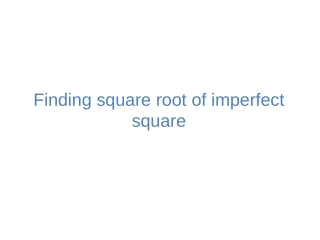DownloadDownload PresentationFinding square root of imperfect square

# Finding square root of imperfect square

Télécharger la présentation## Finding square root of imperfect square

- - - - - - - - - - - - - - - - - - - - - - - - - - - E N D - - - - - - - - - - - - - - - - - - - - - - - - - - -
##### Presentation Transcript

1. Finding square root of imperfectsquare

2. SQUARE ROOT Definition: • When a number is multiplied by itself, the product is called the square of that number. The number itself is called the square root of the product. • It is denoted by √ • For example: The square root of 16 can be written as √16 or (16) ½

3. Recap of Converting Mixed Fractions to Improper Fractions Convert to an improper fraction To convert a mixed fraction to an improper fraction, follow these step Step1 :- Multiply the whole number part by the fraction's denominator. 3 × 5 = 15 Step2 :- Add that to the numerator 15 + 2 = 17 Step 3:- Then write the result on top of the denominator.

4. Example 1: Find the square root of 2 correct to two decimal places 1 .4 1 4 Solution Step 1: Write 2 as decimal number ( up to 4 or 6 decimal points) Step 2: By using long divison Method we can find the Square Root for 2. Thus, √2 = 1.414 1 24 281 2824 2 . 00 00 00 -1 1 0 0 9 6 4 0 0 2 8 1 1 1 9 0 0 1 1 2 9 6 6 0 4 Answer √2 = 1.414 we can round off 2 decimal place√2 = 1.41

5. Example 2:Find the square root of correct to two decimal places 1. 3 0 9 1 23 260 2609 Solution Step 1: Convert given mixed fraction to improper fraction Step 2 : Divide the improper Fractions (upto2 or 4 decimal points). Step 3: Now, find the square root by long division method.  1 . 71 42 00 -1 0 7 1 6 9 2 4 2 0 0 0 2 4 2 0 0 2 3 4 8 1 7 1 9 = 1.7142 Thus, √1.7142 = 1.309 By rounding off 2 decimal place, we have √1.7142= 1.31

6. Try these • Find the square root of 7 correct to two decimal places. • Find the square root of correct to two decimal places. Note: Do not go to the next slide till you finish these sums.

7. Answers • Find the square root of 7 correct to two decimal places. ( 2.65 ) • Find the square root of correct to two decimal places. (1.68 )